• 条件随机场简述1.1 条件随机场定义2. 条件随机场的参数化形式(LinearCRF为例)3. 条件随机场简化形式4. 条件随机场的矩阵形式 1. 条件随机场简述 1.1 条件随机场定义 设X,Y为两个随机变量，若由Y构成的无向图（随机...
目录1. 条件随机场简述1.1 条件随机场定义2. 条件随机场的参数化形式(LinearCRF为例)3. 条件随机场简化形式4. 条件随机场的矩阵形式
1. 条件随机场简述1.1 条件随机场定义
设X,Y为两个随机变量，若由Y构成的无向图（随机变量为结点，变量之间的依赖关系为边）满足全局马尔科夫性（即构成马尔科夫随机场），即
$P(Y_v|X,Y_w:w\neq v) = P(Y_v|X,Y_w : w \sim v )$对有所结点v成立，则条件概率P(Y|X)为条件随机场。
理解：从左至右看就是每个结点基于图中其他结点的条件概率，等于这个结点基于他的相邻结点的条件概率。换句话说就是，每个结点的概率只与他的邻居有关。
线性链条件随机场便是给定的X,Y的长度相同（假设X={x1,x2,…,xn}, Y={y1,y2,…,yn}）是的特殊情况下的条件随机场，为方便计算，我们后面都是以线性链条件随机场为例。
2. 条件随机场的参数化形式(LinearCRF为例)
设P(Y|X)为线性链条件随机场，y,x分别为Y,X的取值，则有：
$P(y|x) = \frac {1}{Z(x)} exp(\sum_{i,k}\lambda_k~t_k(y_{i-1},y_i,x,i) +\sum_{i,l}\mu_l~s_l(y_i,x,i))$
其中 $Z(x)=\sum_y exp(\sum_{i,k}\lambda_k~t_k(y_{i-1},y_i,x,i) +\sum_{i,l}\mu_l~s_l(y_i,x,i))$
我们一个一个来理解。CRF有两个特征：
一个特征是本身的状态, 所以我们有第一个特征函数：$s_l(y_i,x,i)$
一个特征是对前一个状态的依赖，所以我们有第二个特征函数：$t_k(y_{i-1},y_i,x,i)$
$\lambda_k ~,~\mu_l$显然就是每个特征函数对应的权重参数，也是我们要学习的参数。
$exp()$是一个加法乘法转换的常规操作, Z(x)是一个规范化因子，作用是限制P(y|x)为1。
3. 条件随机场简化形式
第一个特征函数为$t_k(y_{i-1},y_i,x,i)$，我们叫它“转移特征”(从i-1转移到i)，设其特征数量为$K_1$
第二个特征函数为$s_l(y_i,x,i)$，我们叫它“状态特征”( i 时刻的状态), 设其特征数量为$K_2$
总特征数量为$K=K_1+K_2$
原来的条件随机场的参数形式有两个特征函数，现在我们将他们合并一个新的特征函数（其实就是直接concate）：
$f_k(y,x) = \sum_{i=1}^{n}f_k(y_{i-1},y_i,x,i)$
其中n为序列的长度
$f_k(y_{i-1},y_i,x,i) = \begin{cases}t_k(y_{i-1},y_i,x,i):k=1,2,...,K_1\\ s_l(y_i,x,i): k=K_1+l,~l=1,2,...,K_2\end{cases}$
相应的，权重也会合并：$w_k=\begin{cases}\lambda_k:k=1,2,...,K_1\\ \mu_l: k=K_1+l,~l=1,2,...,K_2\end{cases}$
合并后的参数和相应权重就构成了新的表达形式：
$P(y|x) = \frac {1}{Z(x)} exp(\sum_{k=1}^{K}w_kf_k(y,x))$
其中
$Z(x)=\sum_y exp(\sum_{k=1}^{K}w_kf_k(y,x))$
我们以$w$表示权重向量，$F(y,x)$表示全局特征向量：
$w = (w_1.w_2,...,w_K)^T$ $F(y,x)=(f_1(y,x),f_2(y,x),...,f_K(y,x))^T$
便可表达成向量内积形式，也就是我们最终的简化形式：
$P_w(y|x) = \frac{exp(w\cdot F(y,x))}{Z_w(x)} ~其中 Z_w(x)=\sum_y exp(w\cdot F(y,x))$
4. 条件随机场的矩阵形式
我们设一个线性链条件随机场$P_w(y|x)$，在长度为n的标记序列y的首尾添加标记$y_0=start,y_{n+1}=end$，现在我们尝试用一个m阶矩阵来表示一个x取值的可能的状态（所以m为所有y可能的取值个数）：
$M_i(x)=[M_i(y_{i-1},y_i |x)]=[exp(W_i(y_{i-1},y_i|x))]=[exp(\sum_{k=1}^Kw_kf_k(y_{i-1},y_i,x,i))]$
这是什么？
给定的观测序列x和标记序列y，$M_i(y_{i-1},y_i|x)$实际上是$y_i$基于x的未规范化的条件概率，也不难得出序列y基于序列x的条件概率：
$P_w(y|x) = \frac {\prod ^{n+1}_{i=1} M_i(y_{i-1},y_i|x)}{Z_w(x)}$
其中$Z_w(x)=(M_1(x)M_2(x)...M_{n+1}(x))_{start,stop}$
参考资料：
统计学习方法 李航


展开全文算法 机器学习 自然语言处理
• 条件随机场 定义 基本问题 1.概率计算问题-向前向后算法 2.学习问题 3.预测问题
条件随机场
定义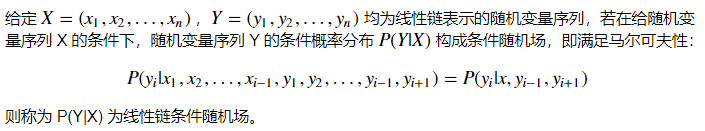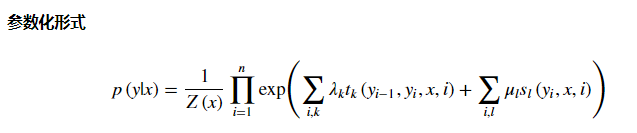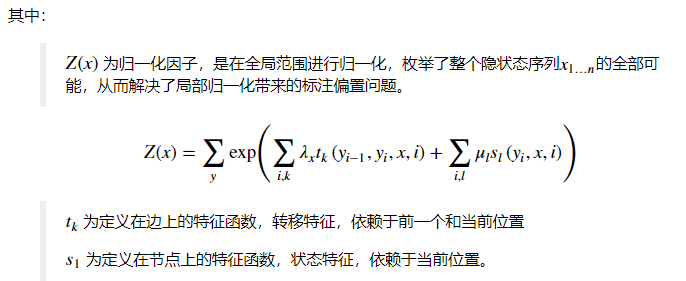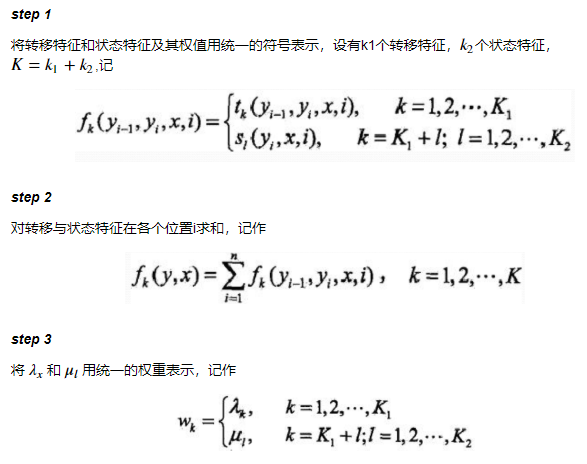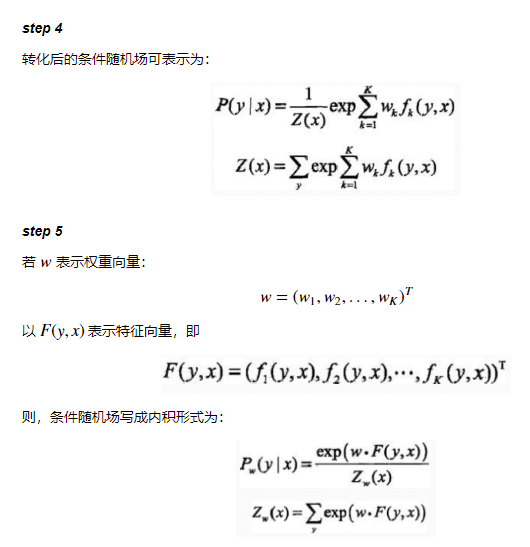基本问题
1.概率计算问题-向前向后算法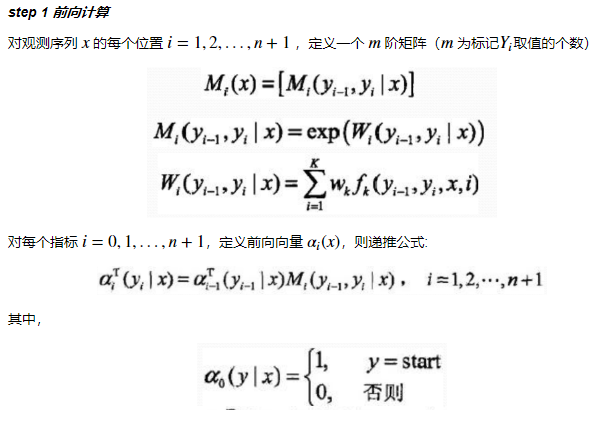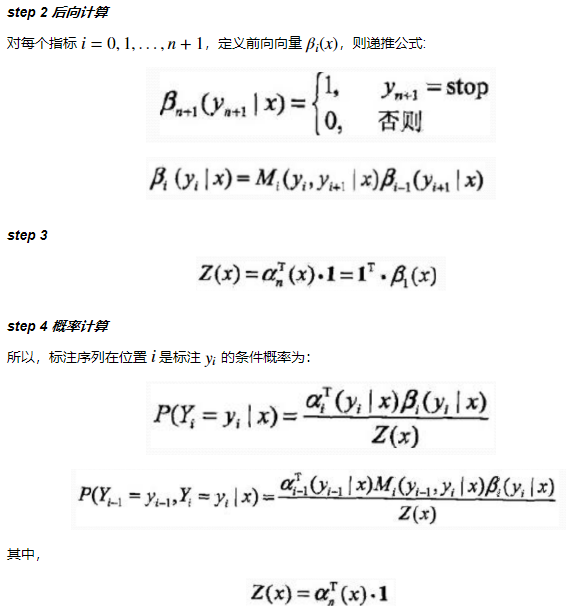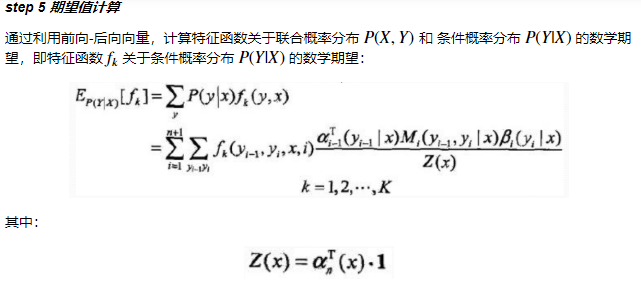2.学习问题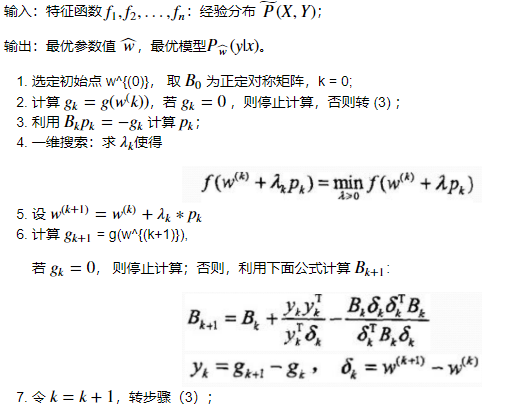3.预测问题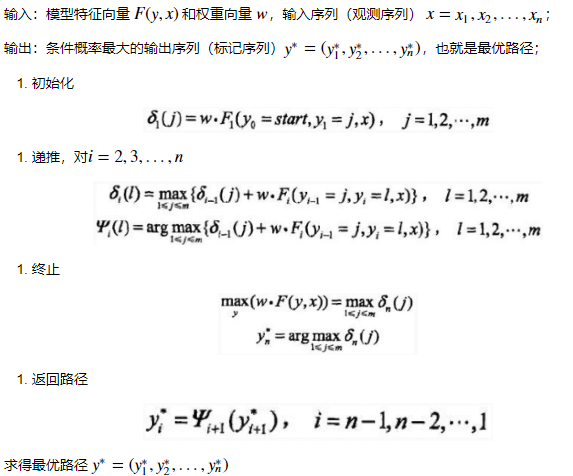展开全文python 算法 机器学习
• 条件随机场定义 条件随机场的参数化形式 条件随机场的简化形式 条件随机场的矩阵形式 转载于:...


2019独角兽企业重金招聘Python工程师标准>>>条件随机场的定义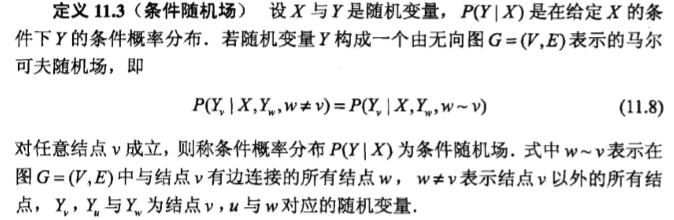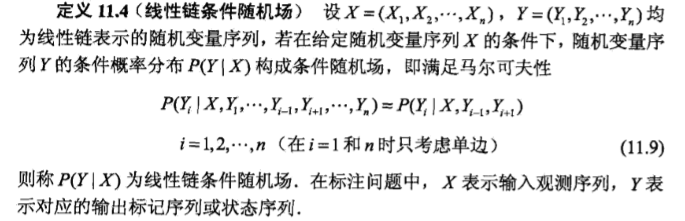条件随机场的参数化形式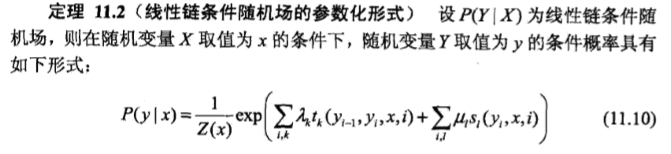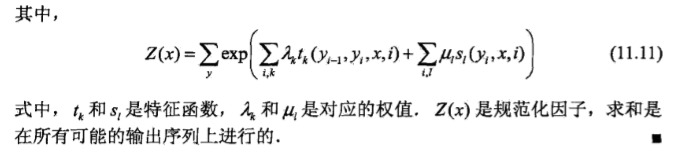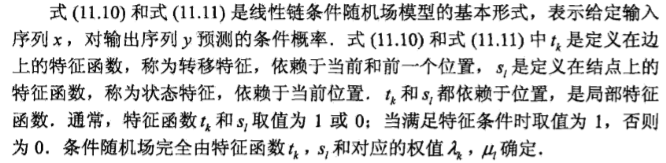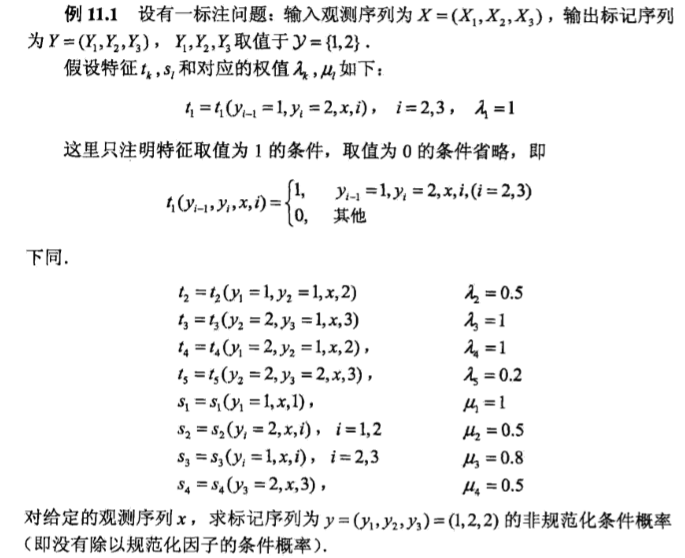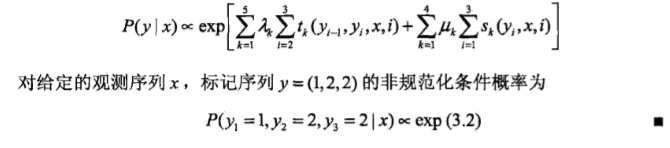条件随机场的简化形式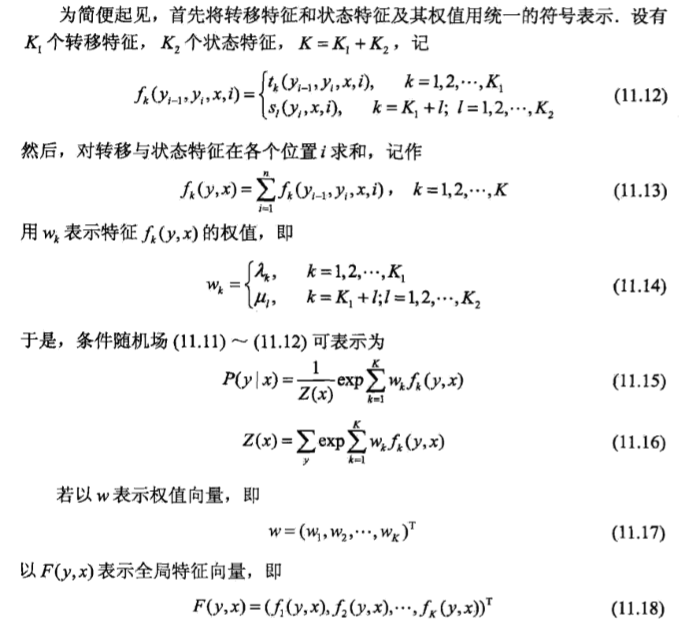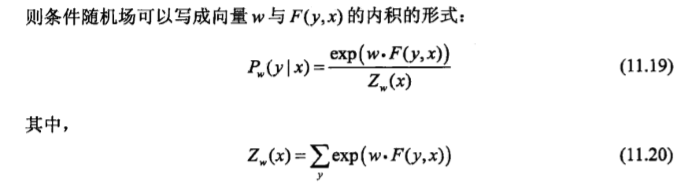条件随机场的矩阵形式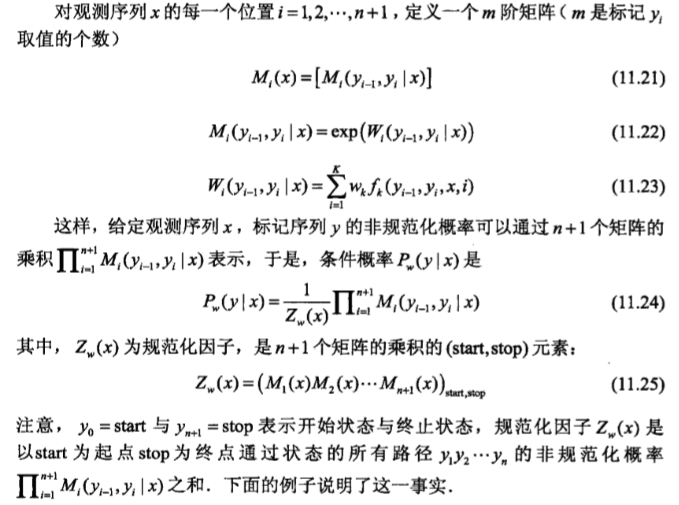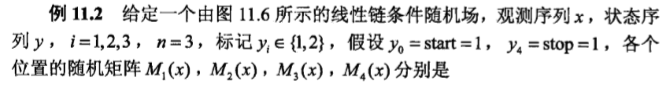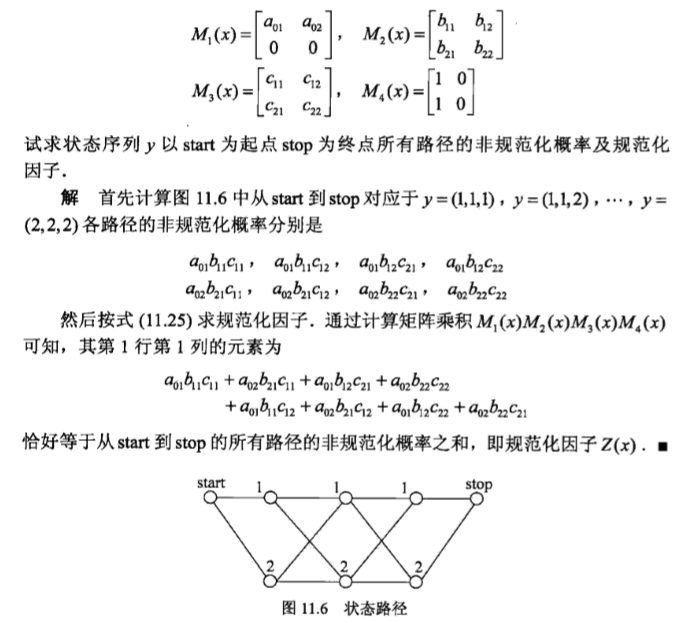转载于:https://my.oschina.net/liyangke/blog/2961465
展开全文• 文章目录1 条件随机场定义1.1 马尔科夫随机场1.1.1 用图模型表示概率1.1.2 局部马尔科夫性1.2 马尔科夫随机场的因子分解 1 条件随机场定义 1.1 马尔科夫随机场 1.1.1 用图模型表示概率 图G=(V,E)，V表示顶点集合，E...
文章目录1 条件随机场定义1.1 马尔科夫随机场1.1.1 用图模型表示概率1.1.2 局部马尔科夫性1.2 马尔科夫随机场的因子分解1.3 条件随机场及线性连条件随机场2条件随机场的表示形式2.1 参数化形式2.2 简化形式2.3 矩阵形式3 条件随机场的概率计算4 最大熵模型4.1最大熵模型定义4.2 最大熵模型学习5 条件随机场的学习算法6 条件随机场的预测算法
1 条件随机场定义
1.1 马尔科夫随机场
1.1.1 用图模型表示概率
图G=(V,E)，V表示顶点集合，E表示边的集合。
概率图模型表示用图表示概率的分布。
可以用无向图G表示联合概率分布P(Y)。Y一定是一个矢量。
顶点$v \in V$表示一个随机变量$Y_v$，$Y = (Y_v)_{v \in V}$。
边$e \in E$表示随机变量之间的概率依赖关系。
1.1.2 局部马尔科夫性
成对马尔科夫性，局部马尔科夫性以及全局马尔科夫性是等价的。
v是无向图G中任意一个结点，对应随机变量$Y_v$。
W是与v有边链接的所有结点，对应随机变量组是$Y_W$。
O是v，W以外的所有结点，对应随机变量组是$Y_O$。
那么 $P(Y_v,Y_O|Y_W) =P(Y_v|Y_W)P(Y_O|Y_W)$，说明给定$Y_W$条件下$Y_v$和$Y_O$是条件独立的。也就是说v只与W有关系。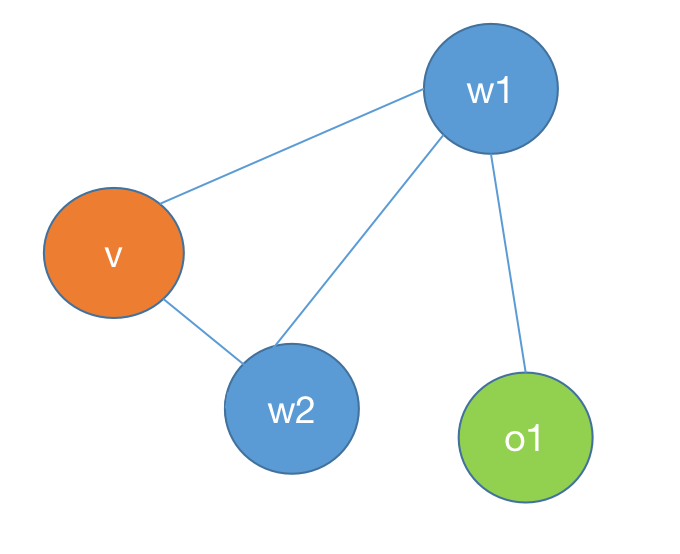如图所示W表示所有与v相连的点。O表示除v和W之外的所有点。
我们再看$P(Y_v,Y_O|Y_W) =P(Y_v|Y_O,Y_W)P(Y_O|Y_W)$(根据条件概率公式)
两个公式联合起来得到结论：$P(Y_v|Y_W) = P(Y_v|Y_O,Y_W)$（记为公式1）
1.2 马尔科夫随机场的因子分解
团：无向图中任意两个结点均有边相连的节点子集。
最⼤团：⽆向图 中的⼀个团，并且不能再加进任何⼀个结点使其成为⼀个更⼤的团。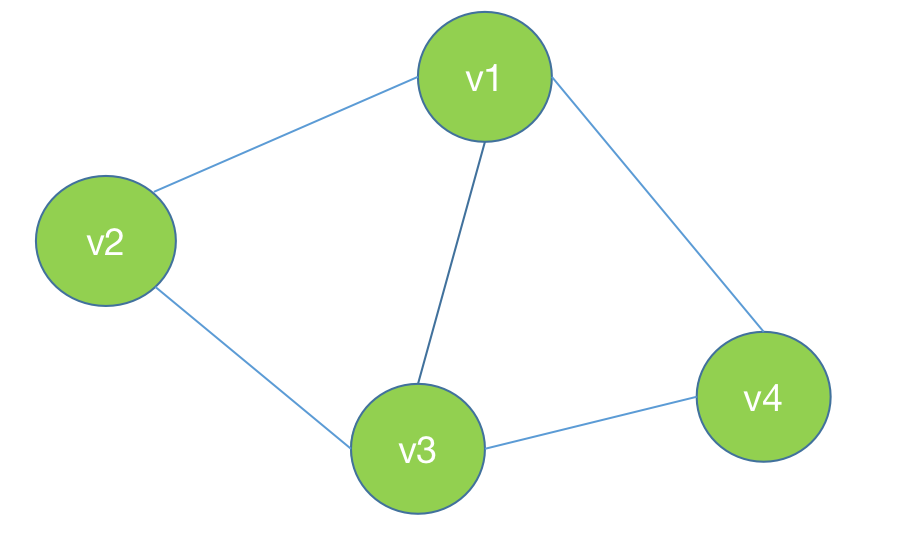这个图中的最大团是(v1,v2,v3)或者(v1,v3,v4)
概率图像图的联合概率分布$P(Y) = \dfrac{1}{Z}\prod_C \Phi_C(Y_C)$
其中C是无向图的最大团，$Y_C$是C的节点对应的随机变量，
势函数$\Phi_C(Y_C)=exp\{-E(Y_C)\}$
Z是规范化因子，是一个全概率分布，$Z=\sum_Y\prod_C\Phi_C(Y_C)$
乘积是在无向图所有的最大图上进行的。
1.3 条件随机场及线性连条件随机场
我们用无向图G表示事件Y的概率，Y中包含Y1,Y2,Y3…Yn。这些事件之间具有线性关系。
再假设还有条件X，X包含X1,X2,X3…Xn。X和Y之间具有线性关系。如下图所示。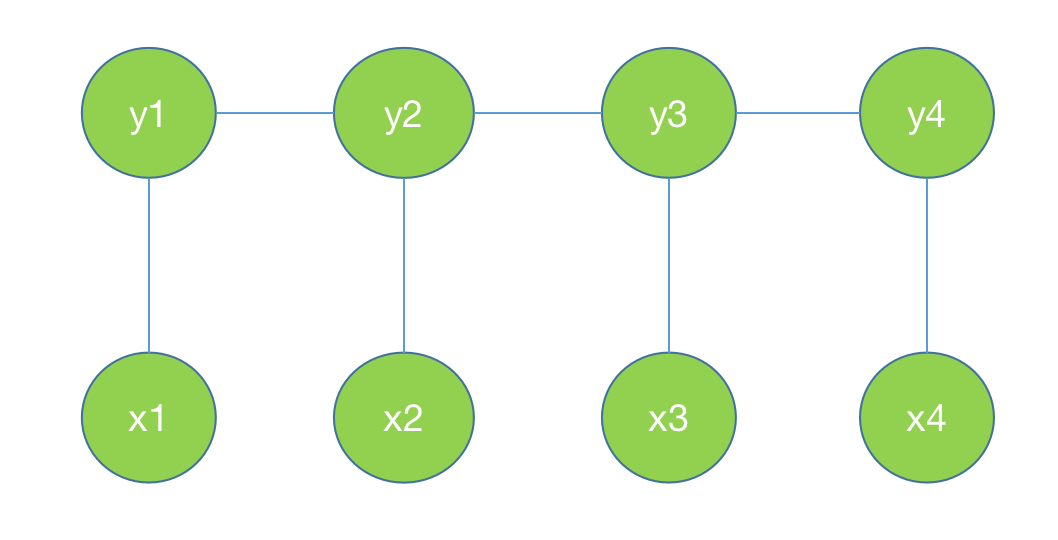那么条件概率P(Y|X)
$P(Y_v|X,Y_w,w\ne v) = P(Y_v|X,Y_w,w-v)$对任意节点v成立，则称条件概率分布P(Y|X)为条件随机场。w-v表示w是与v相连的所有点。也就是说v事件发生的概率只与与它相连的点有关系。
说明：X是Y的条件，X是输入，Y是输出。
这个公式的由来是从公式1对比得到的。
再加上条件Y是具有线性关系的，X和Y具有相同的线性结构。上面的公式可以写为：$P(Y_i|X,Y_1,Y_2...Y_{i-1},Y_{i+1},...Y_n) = P(Y_i|X,Y_{i-1},Y_{i+1})$。这个公式称为线性链条件随机场。记为公式2。
2条件随机场的表示形式
2.1 参数化形式
定义了每个节点和每条边的特征函数，用特征函数表示概率。
$P(Y)=s_1t_1s_2t_2...t_{n-1}s_n$
设P(Y|X)为线性链条件随机场，则在随机变量X取值为x的条件下，随机变量Y取值为y的条件概率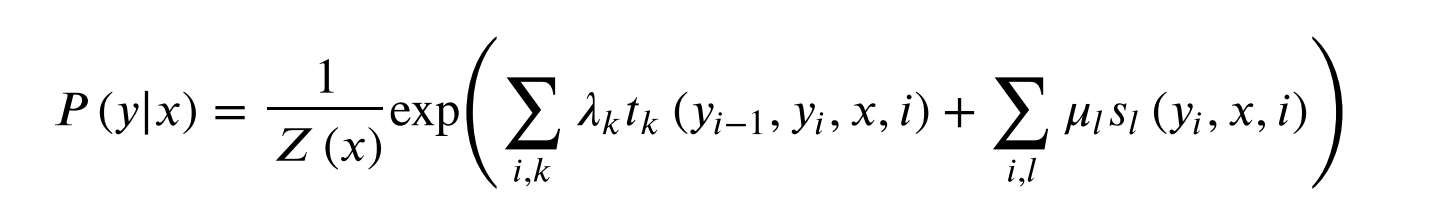其中，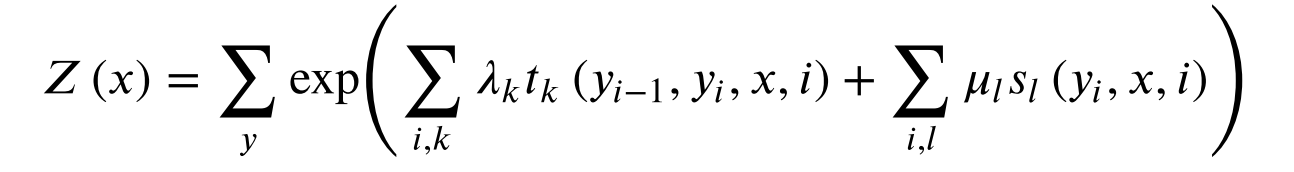$t_k$是定义在边上的特征函数。依赖于当前和前一个位置。
$s_l$是定义在结点上的特征函数，称为状态特征，依赖于当前位置。
$t_k$和$s_l$值为1或者0。
$\lambda_k$和$\mu_l$是对应的权值。
Z(x)是规范化因子，求和是在所有可能的输出序列上。
$s_l$的个数应该等于边的个数每个顶点可能的取值集合个数
$t_k$个数=边的个数（第一个顶点取值个数*第二个顶点取值个数）
2.2 简化形式
用$f_k$表示边的特征函数和节点的特征函数。
设有$K_1$个边的特征函数，有$K_2$个节点的特征函数，$K=K_1+K_2$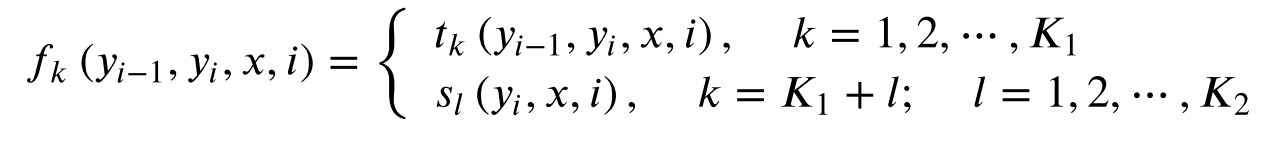用$w_k$表示边的权重和节点的权重。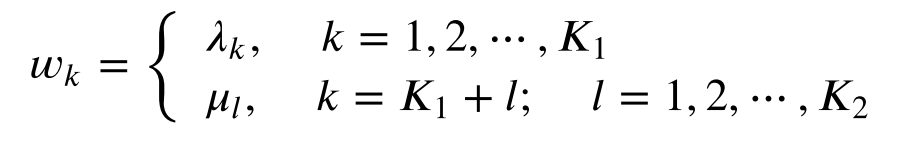则条件随机场表示为：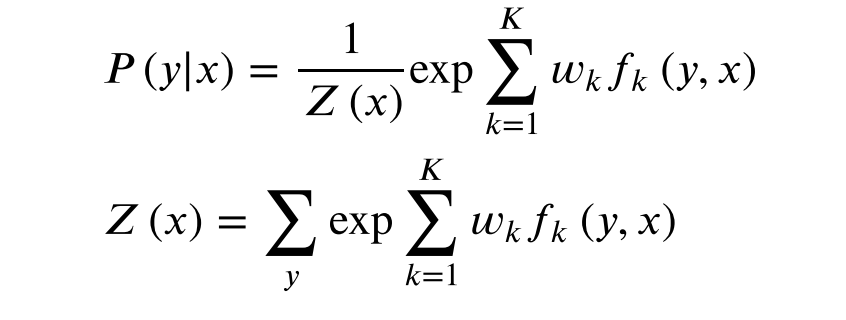用向量化表示为：
$P(y|x)=\dfrac{exp(w.F(y,x))}{Z_w(x)}$，其中
$Z_w(x) = \sum_y exp（w.F(y,x)）$
2.3 矩阵形式
引入一个特殊的起点标记$y_0=start$表示开始状态，$y_{n+1}=stop$表示终止状态。定义一个m阶矩阵。
m是$y_i$取值的个数。
如果$y_i$表示骰子出现的某一面，那么m=6；如果$y_i$表示一枚硬件哪面朝上，那么m=2。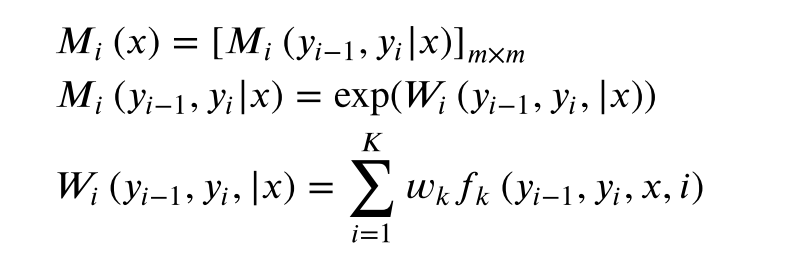$P(y|x) = \dfrac{1}{Z_w(x)}\prod^{n+1}_{i=1}M_i(y_{i-1},y_i|x)$，
其中$Z_w(x)=(M_1(x),M_2(x),...M_{n+1}(x))_{start,stop}$
矩阵最关注矩阵的形状。
3 条件随机场的概率计算
前向计算
后向计算
计算特征函数$f_k(x,y)$关于条件分布P(Y|X)的数学期望：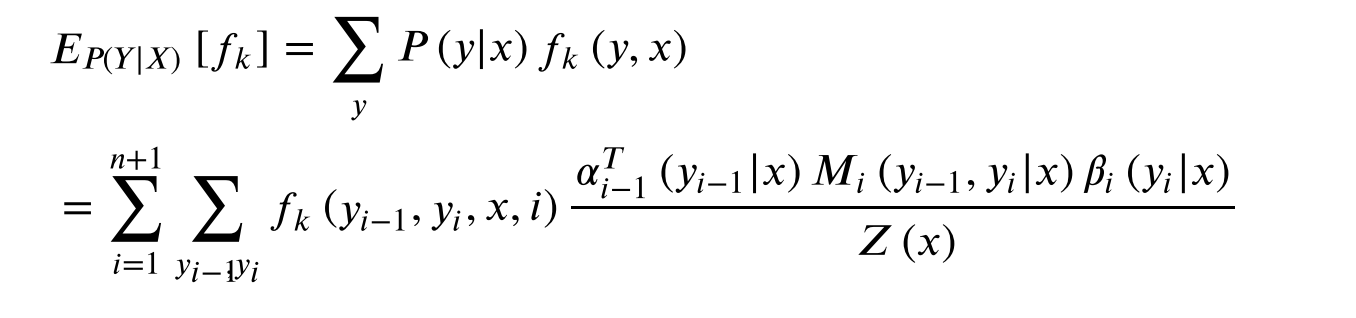计算特征函数$f_k(x,y)$关于联合分布P(X,Y)的数学期望：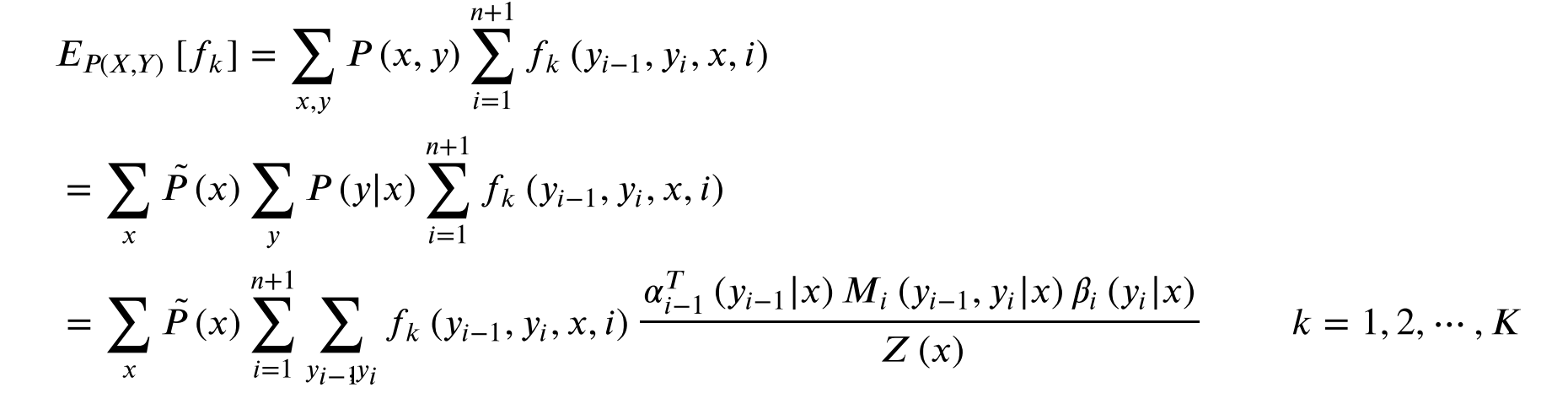这里的结果是中间量
4 最大熵模型
4.1最大熵模型定义
模型就是一个从输入到输出的一个映射，可以是一个f(x)，也可以是一个P(y|x)。当在所有条件都满足的时候，这个函数不唯一的时候，就使用最大熵策略来选择模型。所以最大熵模型是一种选择策略，是一种世界观。
熵最大=变量几乎可以均匀分布
假设分类模型是条件概率分布P(Y|X)。给定条件X，以条件概率P(Y|X)输出Y。
给定训练集，可以确定联合分布P(X,Y)的经验分布和边缘分布P(X)的经验分布，分别以$\hat{P}(X,Y)$和$\hat{P}(X)$。这里
$\hat{P}(X=x,Y=y)=\dfrac{v(X=x,Y=y)}{N}$
$\hat{P}(X=x)=\dfrac{v(X=x)}{N}$
其中，$v(X=x,Y=y)$表示训练样本中(x,y)出现的频率，$v(X=x)$表示训练样本中x出现的频率。N表示样本容量。
特征函数f(x,y)描述输入x和y之间的某一事实。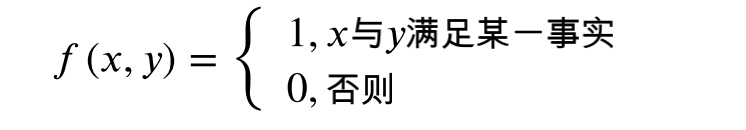特征函数f(x,y)关于经验分布$\hat{P}(X,Y)$的期望:
$E_{\hat{P}}(f)=\sum_{x,y}\hat{P}(x,y)f(x,y)$
特征函数f(x,y)关于模型P(Y|X)与经验分布$\hat{P}(X)$的期望
$E_{P}(f)=\sum_{x,y}\hat{P}(x)P(y|x)f(x,y)$
假设：特征函数f(x,y)关于经验分布$\hat{P}(X,Y)$的期望应该等于关于模型P(Y|X)与经验分布$\hat{P}(X)$的期望。
最⼤熵模型：假设满⾜所有约束条件的模型集合为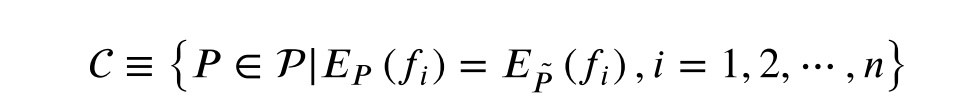定义在条件概率分布P(Y|X)上的条件熵为：
$H(P)=-\sum_{x,y}\hat{P}(x)P(y|x)logP(y|x)$
4.2 最大熵模型学习
优化函数：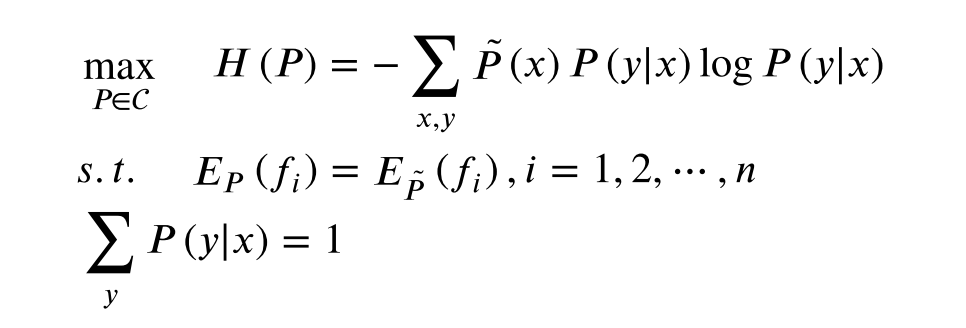拉格朗日乘子法
1 构建拉格朗日乘子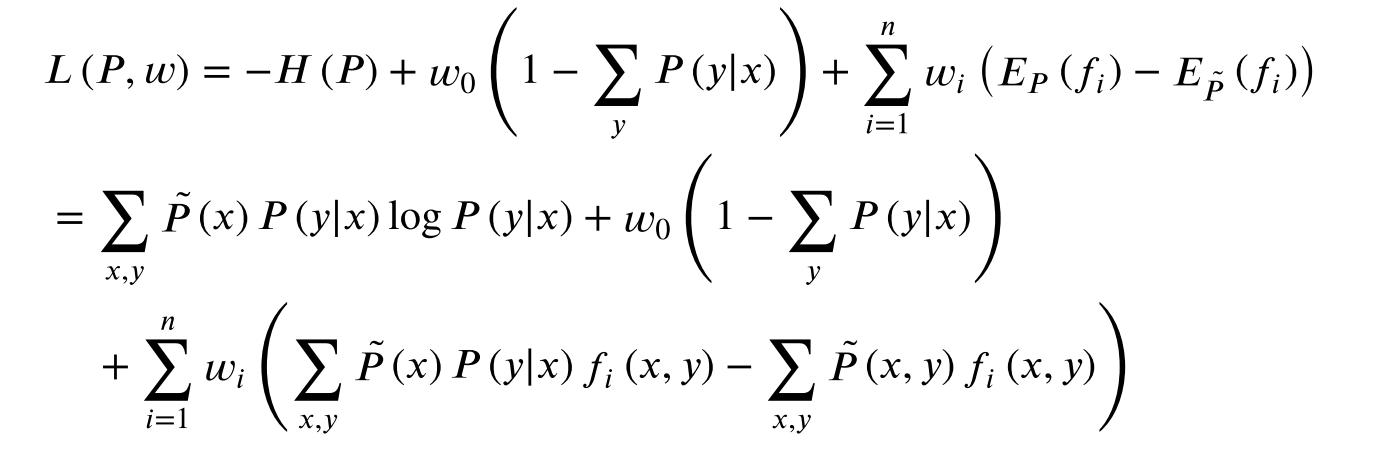2 求min
3 求max
最⼤熵模型的极⼤似然估计等价于对偶函数极⼤化。
对偶函数如下：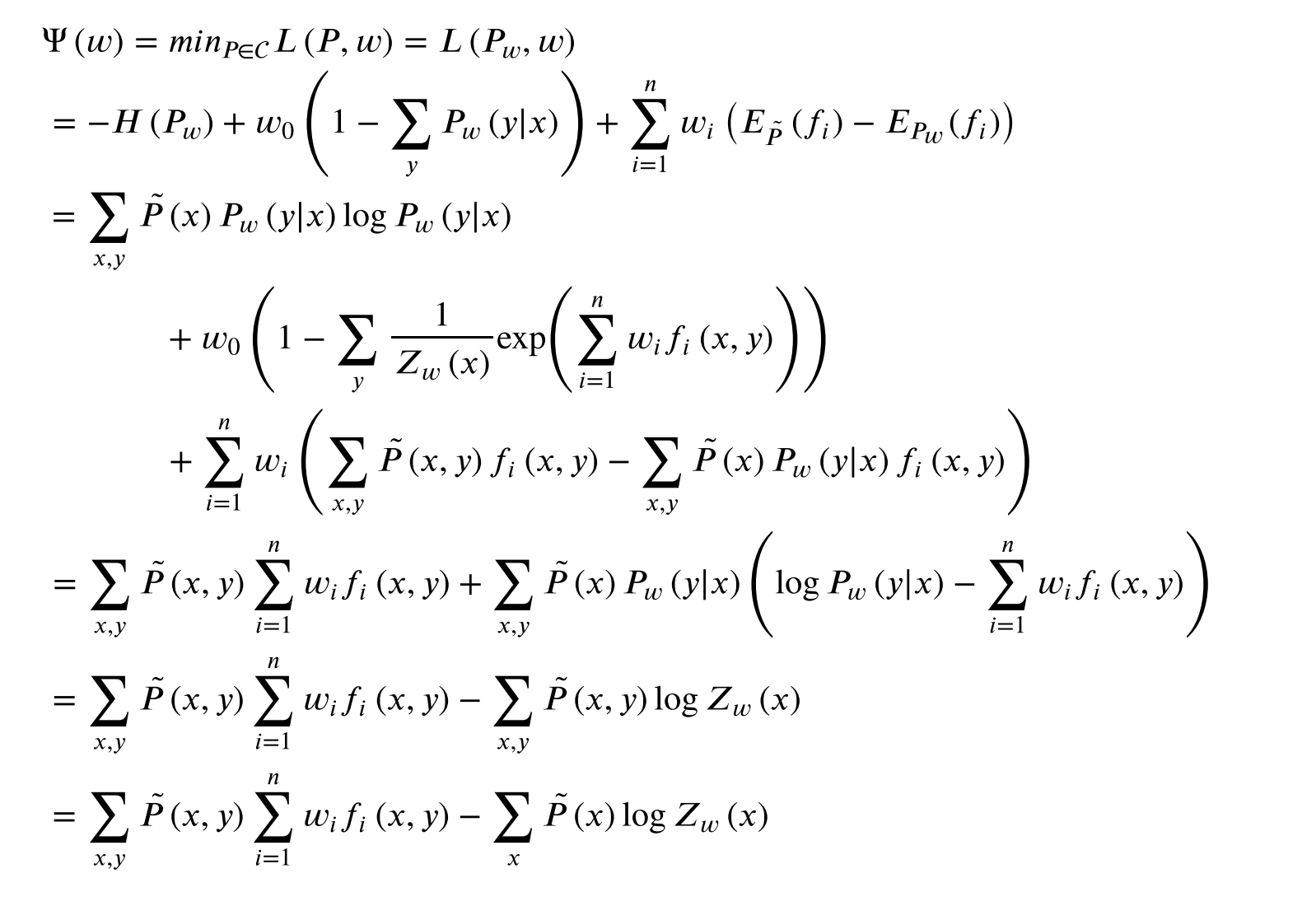4 模型学习：迭代尺度法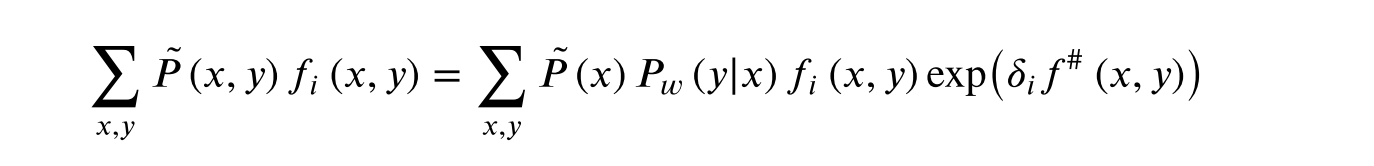这部分的学习跳过了。直接用结论。
5 条件随机场的学习算法
由训练数集，计算经验概率分布$\hat{P}(X,Y)$。
目标函数是对数似然函数。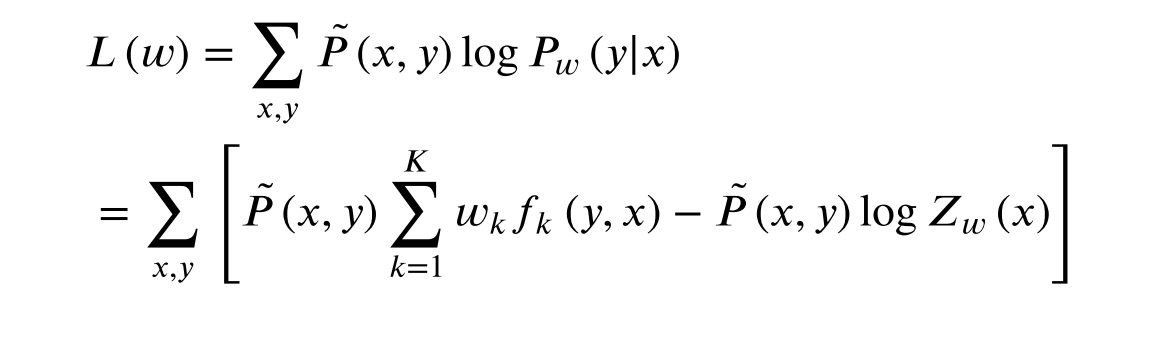6 条件随机场的预测算法
给定条件随机场P(Y|X)和输入观测序列x，求条件概率最大的标记序列y*。
公式太多，没有记录。


展开全文• ## 条件随机场 CRF

千次阅读 2018-04-27 09:46:40
随机变量 Χ 表示需要标记的观察序列集。随机变量 Υ表示相应的表示标记序列集。所有的 Υ i ∈ Υ 被假设在一个大小为 N 的有限字符集内。... 条件随机场定义:令 G = ( V , E ) 表示一个无向图, Υ = ...
• 本文仅仅讲解一下马尔可夫随机场和条件随机场定义和形式，不会涉及到条件随机场的概率计算和模型学习问题，因为阅读的书籍和博客都讲的很混乱，所以深入的讲解很难进行，望见谅。 一、马尔可夫随机场 马尔可夫随机...
• 本文主要介绍定义在线性链上的特殊的条件随机场，称为线性链条件随机场（linear-chain CRF)。线性链条件随机场可以用于机器学习里的标注问题。这时，在条件概率模型 P(Y|X)P(Y|X) 中，Y 是输出变量，表示标记...机器学习
• 第一部分给出概率图模型的定义与性质，以及对它意义重大的因子分解定理；第二部分给出条件随机场本质是概率图模型这一定义，而后针对使用最多的线性链条件随机场给出概率计算以及参数学习算法。
• 条件随机场模型实际上是定义在时序数据上的对数线形模型，其学习方法包括极大似然估计和正则化的极大似然估计。具体的优化实现算法有改进的迭代尺度法IIS、梯度下降法以及 L-BFGS 算法。（crf++ 采用了 L-BFGS 优化...
• 条件随机场 定义1： 条件随机场（conditional random field，简称CRF）是一种判别式无向图模型。生成式模型是直接对联合分布进行建模，而判别式模型则是对条件分布进行建模，隐马尔可夫模型就是生成式模型。——...CRF 词性标注
• 条件随机场(CRF)是给定一组输入随机变量X的条件下另一组输出随机变量Y的条件概率分布模型，其特点是假设输出随机变量构成马尔科夫随机场。实际上是定义在时序数据上的对数线性模型。条件随机场属于判别模型...
• 1. 条件随机场定义 1.1 定义 条件随机场(conditional random field, CRF)是给定一组输入随机变量条件下另一组输出随机变量的条件概率分布模型,其特点是假设输出随机变量构成马尔可夫随机场.CRF可以用于不同的预测...人工智能
• ## 条件随机场的简单理解

万次阅读 多人点赞 2018-09-07 17:24:05
条件随机场总的来说就是只要满足“条件随机场”这个条件，就可以根据定义的模型去求解我们需要求解的问题，而我们时长需要解决的问题以线性的居多，所谓线性就是满足“线性链条件随机场”，本文也只涉及对“线性链条...CRF 自然语言处理 nlp
• 条件随机场定义：给定随机变量X（观测序列）条件下，随机变量Y（状态序列）的马尔可夫随机场。  （由此可以看出，条件随机场是条件概率分布问题，马尔可夫随机场是联合概率分布问题。）    已知马尔可夫...统计学
• 1.3 条件随机场的参数化形式 1.4条件随机场对应的简化概率表达 2. 例子 定义CRF中的特征函数 从特征函数到概率 CRF与逻辑回归的比较 CRF与HMM的比较 HMM和CRF区别 3. Tensorflow实现 tf.contrib.crf （1...CRF
• 小白学习：李航《统计...目录小白学习：李航《统计学习方法》第二版第11章 条件随机场（二）----条件随机场前言一、条件随机场定义与形式条件随机场定义线性链条件随机场线性链条件随机场的定义举个栗子线性链条件算法 机器学习 统计模型 计算机视觉
• 本篇紧接上篇笔记(总结)-从马尔可夫模型到条件随机场-2，讲述条件随机场（Conditional Random Field）。 条件随机场 定义 先给出几个相关概念的定义。 随机场:可看成是一组随机变量的集合(这组随机变量...概率图模型 序列标注
• ## 条件随机场（CRF）

千次阅读 2020-10-30 09:34:09
2.1 条件随机场的参数化形式 2.2 条件随机场的简化形式 2.3 条件随机场的矩阵形式 3.概率计算问题 3.1 前向-后向算法 3.2 概率计算 3.3 期望值计算 4.学习算法（参数估计） 5.预测算法（.
• 写在前面 前面写完了HMM，比较重点的就是HMM的三个问题，需要好好消化。这篇博客主要介绍条件随机场，相比于HMM，CRF的应用可能会更广。从刚接触CRF开始也很久了...条件随机场定义 首先给出来自小蓝书的CRF定义： ...
• ## 条件随机场(CRF) - 2 - 定义和形式

万次阅读 多人点赞 2016-05-25 15:46:58
书上首先介绍概率无向图模型，然后叙述条件随机场定义和各种表示方法，那这里也按照这个顺序来。 概率无向图模型(马尔可夫随机场) 其实这个又叫做马尔可夫随机场(MRF)，而这里需要讲解的条件随机场就和其有脱不开...算法 机器学习...